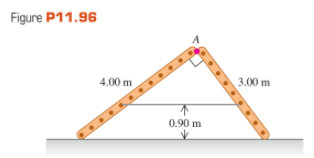×
Get Full Access to University Physics - 13 Edition - Chapter 11 - Problem 96cp
Get Full Access to University Physics - 13 Edition - Chapter 11 - Problem 96cp

×

# Two ladders, 4.00 m and 3.00 m long, are hinged at point AISBN: 9780321675460 31

## Solution for problem 96CP Chapter 11

University Physics | 13th Edition

• Textbook Solutions
• 2901 Step-by-step solutions solved by professors and subject experts
• Get 24/7 help from StudySoup virtual teaching assistantsUniversity Physics | 13th Edition

4 5 1 426 Reviews
26
1
Problem 96CP

Two ladders, 4.00 m and 3.00 m long, are hinged at point A and tied together by a horizontal rope 0.90 m above the floor (Fig. P11.96). The ladders weigh 480 N and 360 N, respectively, and the center of gravity of each is at its center. Assume that the floor is freshly waxed and frictionless.

(a) Find the upward force at the bottom of each ladder.

(b) Find the tension in the rope.

(c) Find the magnitude of the force one ladder exerts on the other at point A.

(d) If an 800-N painter stands at point A, find the tension in the horizontal rope.Step-by-Step Solution:

Step 1 of 8

Given data:

*Length of the two ladders,and*The ladders are tied together by a horizontal rope,*Weight of ladders areandStep 2 of 8

Step 3 of 8

##### ISBN: 9780321675460

The answer to “?Two ladders, 4.00 m and 3.00 m long, are hinged at point A and tied together by a horizontal rope 0.90 m above the floor (Fig. P11.96). The ladders weigh 480 N and 360 N, respectively, and the center of gravity of each is at its center. Assume that the floor is freshly waxed and frictionless. (a) Find the upward force at the bottom of each ladder. (b) Find the tension in the rope. (c) Find the magnitude of the force one ladder exerts on the other at point A. (d) If an 800-N painter stands at point A, find the tension in the horizontal rope.” is broken down into a number of easy to follow steps, and 106 words. University Physics was written by and is associated to the ISBN: 9780321675460. Since the solution to 96CP from 11 chapter was answered, more than 1245 students have viewed the full step-by-step answer. This textbook survival guide was created for the textbook: University Physics, edition: 13. This full solution covers the following key subjects: Find, point, rope, ladders, tension. This expansive textbook survival guide covers 26 chapters, and 2929 solutions. The full step-by-step solution to problem: 96CP from chapter: 11 was answered by , our top Physics solution expert on 05/06/17, 06:07PM.

Unlock Textbook Solution